Latest Banking jobs   »

# Quantitative Aptitude Quiz For NABARD Grade A 2022- 3rd August

Q1. A sold an article to B at 30% profit and B further sold it to C at 10% loss. C then sold it to D at 40% profit. If difference of cost price of article for D & B is Rs.169, then find the amount at which C sold the article to D? (in Rs.)
(a)550
(b)819
(c)650
(d)585
(e)850

Q2. A container contains mixture of 200 lit of pure milk and ‘P’ liters of water. Ratio of 40% of mixture to the quantity of water is 6 : 5 and when Q liters of water is added to mixture, then ratio of total mixture to water becomes 15 : 7. Find the value of P-Q?
(a)40
(b)50
(c)25
(d)30
(e)20

Q3. Pipe A, B & C alone can fill the tank in 15, 12 & 10 min respectively. If Pipes are opened in such a way that for the 1st min pipe A & B together are opened, in 2nd min pipe B & C min are opened, in 3rd min pipe C & A are opened and so on for next coming min, then find the time to completely filled the tank? (in min)
(a)11.5
(b)15
(c)12
(d)6
(e)9

Q4. Surface area of a sphere is 5544 sq.m. Find the curved surface area of cylinder (sq.m.) if the sphere is melted to form a cylinder of height 28/9 m?
(a) 1232
(b) 1276
(c) 1122
(d) 1176
(e) 1342

Q5. Reena deposited two parts of a sum of Rs.32000 in different banks at the rates of 12% per annum and 20% per annum respectively at SI. In one year she got Rs.4850 as the total interest. What was the amount deposited at the rate of 12% per annum? (in Rs.)
(a)19950
(b)20850
(c)21875
(d)21115
(e)19375

Q6. The average price of a phone, laptop & printer is Rs.8000 less than average price of phone & laptop. The amount by which the price of phone is more than that of laptop is same as amount by which price of printer is less than that of laptop. Find difference between average price of phone & laptop and average price of laptop & printer? (in Rs.)
(a)8000
(b)12000
(c)16000
(d)20000
(e)24000

Q7. The ratio of speed of boat in downstream to upstream is 9 : 5 and total time taken by boat to cover 105 km in still water in 3 hours. Find the difference in total time taken by boat to cover 225 in downstream and to that in upstream? (in hours)
(a)4
(b)2.5
(c)7.5
(d)5
(e)8

Q8. P and Q started a business by investing Rs.40,000 and Rs.32,000 respectively. After four months R joined the business with a capital of Rs.48,000. After two more months Q left the business. At the end of the year, difference between profit share of P and Q is Rs.6600. What is the total profit earned? (in Rs.)
(a)21800
(b)23000
(c)29800
(d)27600
(e)24200

Q9. If 6 boys take 1.5 days less than time taken by ‘n’ number of men to complete a task and work done by four men in one day is same as the work done by six boys in one day. Moreover, n men complete task in 6 days. Find the value of n.
(a)5
(b)2
(c)6
(d)3
(e)4

Q10. A man invested an amount in the ratio of 4: 3 at the rate of (R/2-2.5)% & (R – 6)% respectively at simple interest. If bigger amount invested for 3 years and smaller amount invested for 4 years and man gets interest in the ratio of 5 :8, then find the value of R?
(a)20%
(b)10%
(c)15%
(d)30%
(e)25%

Q11. Find the number of blue balls in the bag if the bag contains ‘x’ red balls & ‘y’ blue balls such that x²+4x-12=0 and probability of drawing a red ball from the bag at random is 2/5?
(a)3
(b)2
(c)4
(d)5
(e)1

Q12. The difference of present age of A and B is 15 years. The ratio of A’s age 4 years ago and B’ age 2 years hence is 4:1, then find the sum of their ages after 6 years? (in years)
(a)22
(b)29
(c)33
(d)39
(e)46

Q13. Priya marks up a phone 25% above its cost price and after allowing two successive discounts of 12% and 15% suffers a loss of Rs. 195. Find the difference between the cost price and marked price of the phone. (in Rs.)
(a) 1250
(b) 1000
(c) 750
(d) 500
(e) 950

Q14. A man invested Rs. P on simple interest at the rate of 10% per annum for 3 years. He invested the earned interest on compound interest for 2 years at the same rate and earned interest of Rs.157.50. Find the value of P/50-4²?
(a) 48
(b) 34
(c) 52
(d) 60
(e) 66

Q15. Train ‘A’ can cross a platform of 285 m long in 27 sec and takes 16 sec to completely cross another train B coming from opposite direction of twice the length of train A. If speed of train B is 50% less than that of train A, then find the speed of train A? (in m/s)
(a) 21
(b) 9
(c) 12
(d) 15
(e) 18

Solutions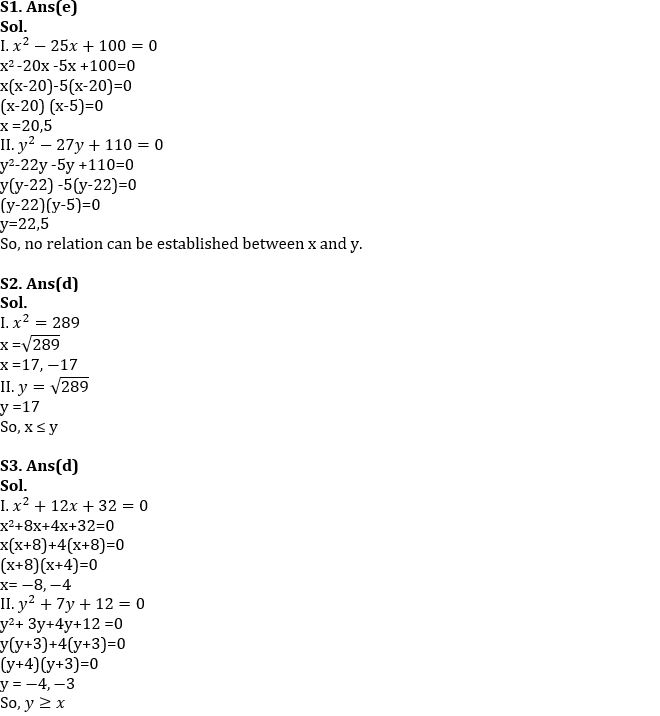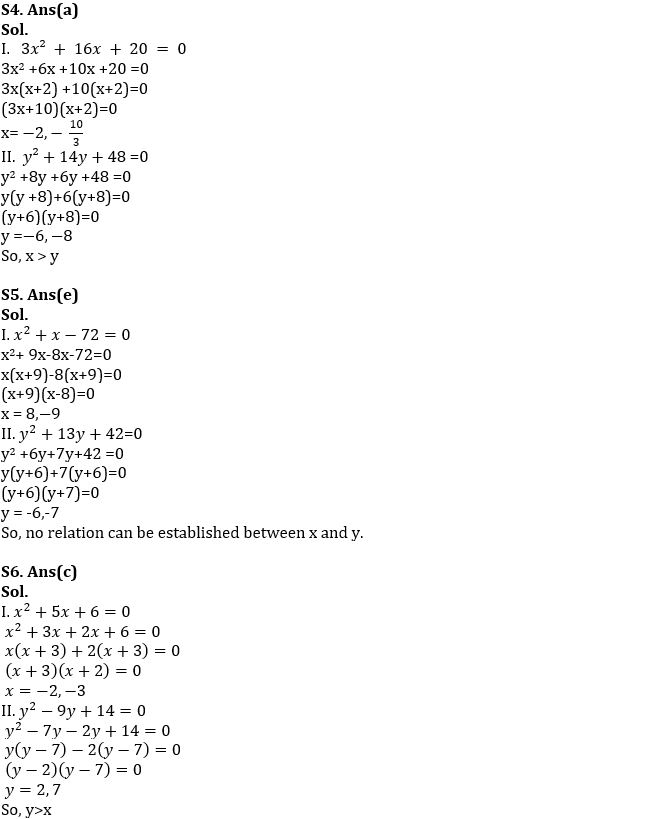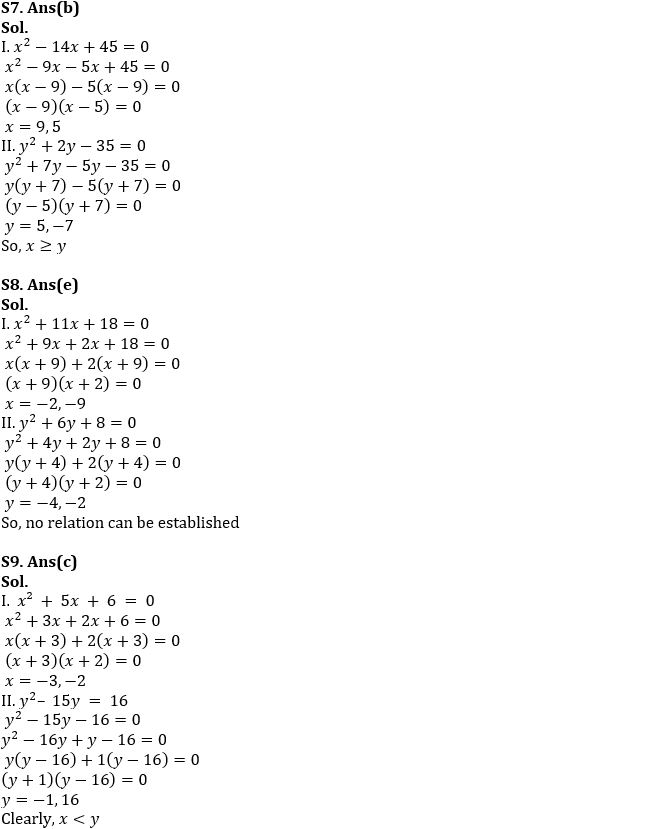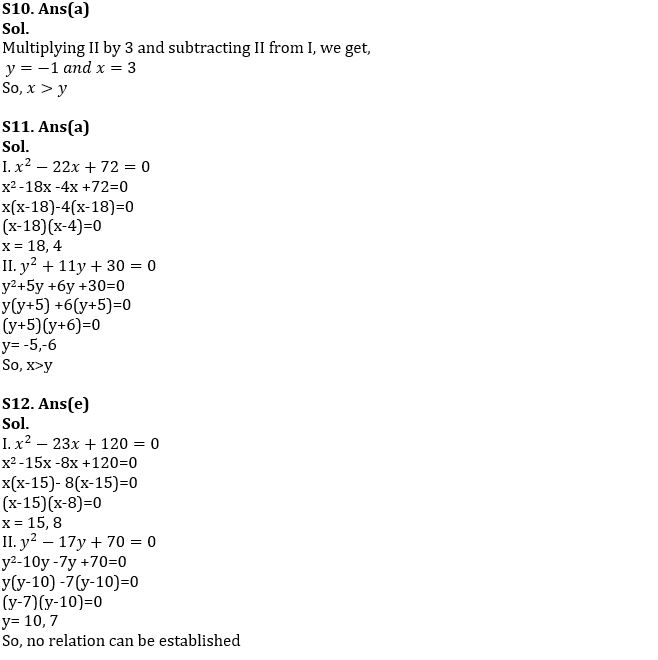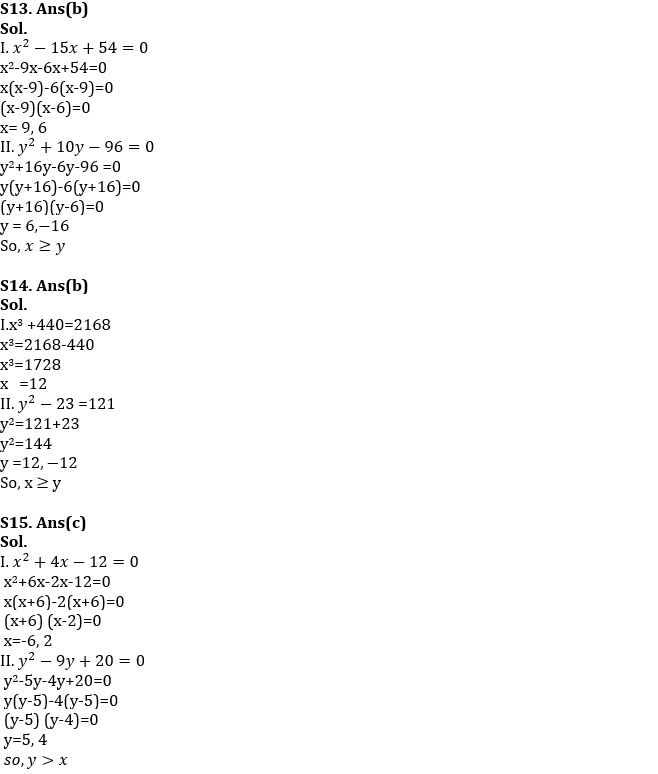#### Congratulations!Incorrect details? Fill the form again here

•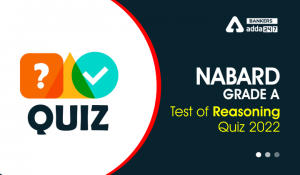Reasoning Ability Quiz For NABARD Grade ...
•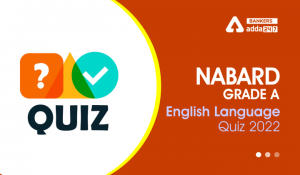English Quizzes For NABARD Grade A 2022-...
•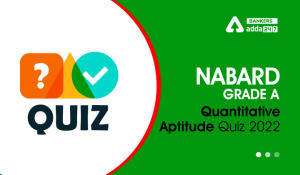Quantitative Aptitude Quiz For NABARD Gr...
•Reasoning Ability Quiz For NABARD Grade ...
•English Quizzes For NABARD Grade A 2022-...
•Quantitative Aptitude Quiz For NABARD Gr...